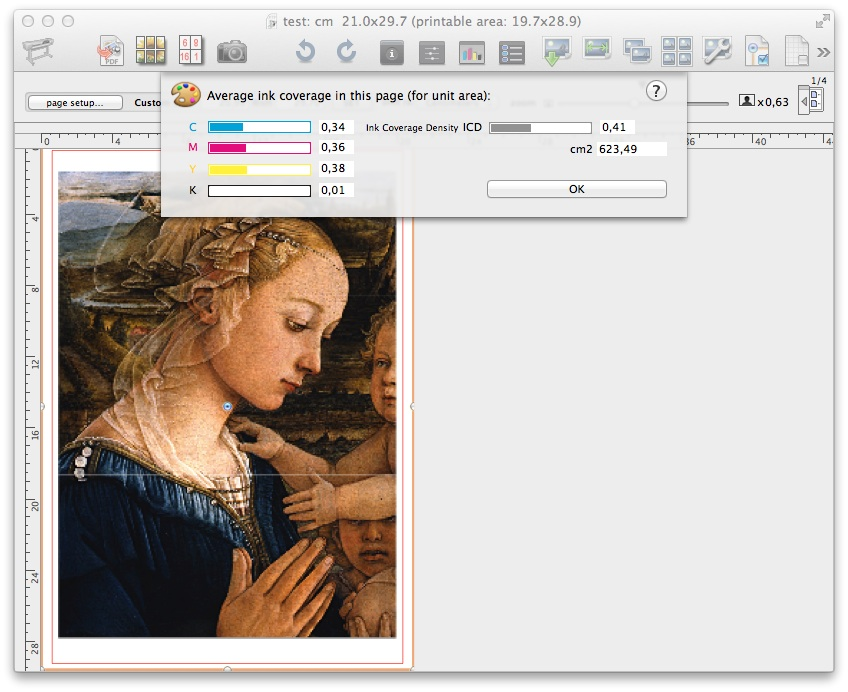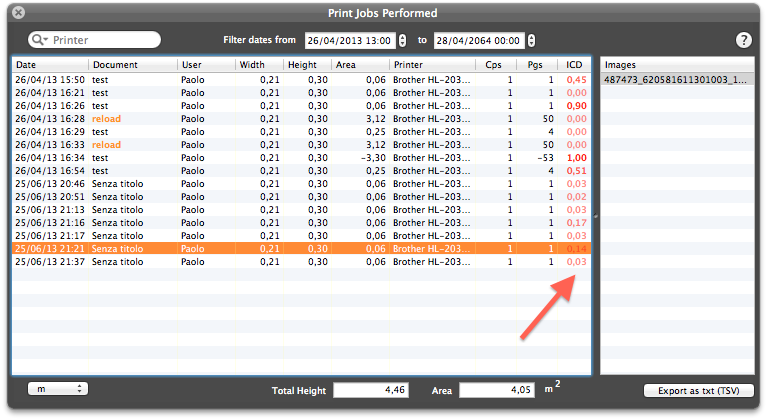### ICD (ink coverage density)

Another unique feature available in FitPlot is the ink coverage density (ICD), useful for print shops and home users to evaluate print costs.
Requesting this function (menu View -> Ink Coverage Density [cmd-shift-I]) brings up a little modal panel that shows the average density of inks in the currently showed page.
You can either call this feature by clicking on thetoolbar button (needs you set up manually your toolbar to show this button, see how to set up the toolbar here).Ink coverage is calculated separately on CMYK basis (generic CMYK profile) and on gray scale, with range values [0-1]. The gray scale value is used as ink coverage density [ICD] throughout the app.

ICD testify the ink coverage per square unit (density) in a range from 0 (no ink) to 1 (100% coverage of all CYMK components), thus you could use ICD value to have an immediate evaluation of print costs.

When you use ICD you can estimate your effective cost more accurately than ever. FitPlot will help you to determine print prices based on paper type, paper size, print quality and color density.

It's up to you to obtain the cost (constant) to ascribe per square unit, in way that the analytic price is so calculated:

``` ICD x ink cost per sq. unit x printed surface + paper cost per sq. unit x printed surface = Price ```

then simplifying:

``` (ICD x ink cost per sq. unit + paper cost per sq. unit) x printed surface = Price ```

The paper cost per square unit is easy to find:
Paper roll 80 gr/mq, 91,4 cm (42")x50m is priced about 15€, so let's calculate what is the cost per cmq (because this is the unit for our next calculations).

`Total area of the roll is: 91,4x500 = 45700 cmq`

`paper cost per cmq is: 15 / 45700 = 0,00032822757112 € / cmq`

Now that we have the paper, let us see an example with an hypothetical ink unit price of 0,003 €/cmq. Note that this price has been guessed from rates seen on the web, so take it as a sample. The same words are to say about the paper price.

##### Table of calculated costs

ink price €/cmq paper price €/cmq page size = A0 surface cmq ICD content total €
0.003 0.00033 84.1x118.9 cm 9,999.49 0.80 dark photo 27.30
0.50 medium photo 18.30
0.20 cad with filled areas 9.30